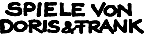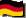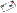Great German Games with a HedgehogOur Games About us Online Games Events Other Contact Order Infos Home  >  Online Games  >  Numfield  >  Evaluation Class# Numfield, an Evaluation Class

The following piece of Java Source code has been contributed byDon Reble . It contains a complete class for efficent and elegant scoring of turns. Have a look at it. If anyone wants to use it for his strategy, I will put it in the standard environment.
 ```/* This class calculates one's profit, if one had made different bets during a turn. In principle, it is the same as Shark5's eval() function, but runs faster, and is sometimes easier to use. */ public class Evaluator { /* ----- data ----- */ private int d_bonusAndCarry; // The bonus plus carry-over. /* For our purposes, we define "bettor" as an _opponent_ who bet more than zero. That is, a zero-bettor is not a bettor, and I am not a bettor (because I am not my opponent). */ private int d_bettors; // The number of bettors. private int[] d_betHistogram; // BetHistogram[N] has the number of bettors who bet // between 1 and N (inclusive). private int d_totalBet; // The sum of all bettor's bets. private int d_totalShares; // The shares of a bettor is the number of bettors who did not // bet more than he. A bettor must have at least one share, // since he did not bet more than himself. The sum of all // bettors' shares is the Total Shares. /* ----- constructor ----- */ public Evaluator(int bonusAndCarry, int bets[]) { // bets is my bet, which is ignored throughout. d_bonusAndCarry = bonusAndCarry; /* -- Find the largest bettor's bet, and allocate the histogram. -- */ int maxbet = 0; for (int ix = 1; ix < bets.length; ix += 1) { int bet = bets[ix]; if (bet > maxbet) maxbet = bet; } d_betHistogram = new int[maxbet+1]; /* -- Accumulate the non-zero bets. -- */ // Also, count the bettors, and sum their total bet. // Temporarily, histogram[N] counts the bettors who bet exactly N. d_bettors = 0; for (int ix = 1; ix < bets.length; ix += 1) { int bet = bets[ix]; if (bet > 0) { d_betHistogram[bet] += 1; d_bettors += 1; d_totalBet += bet; } } /* -- Compute the total shares. -- */ // Also, cumulate the histogram. // Compute the total shares, assuming no equal bets. d_totalShares = ((d_bettors + 1) * d_bettors) / 2; // Adjust total shares for equal bets. int sum = 0; for (int bx = 1; bx <= maxbet; bx += 1) { int matches = d_betHistogram[bx]; d_totalShares += ((matches - 1) * matches) / 2; sum += matches; d_betHistogram[bx] = sum; // cumulating the histogram } } public int gain(int mybet) { /* -- Compute the gain I would have when betting Mybet. -- */ // The histogram makes this a constant-time function. if (mybet == 0) return 0; // Include my bet and the bonus in the stake. int stake = d_totalBet + d_bonusAndCarry + mybet; // Compute my shares, and the number of players I match. int myMatches = 1; int myShares = 1; if (mybet >= d_betHistogram.length) { myShares += d_bettors; } else { myShares += d_betHistogram[mybet]; myMatches += d_betHistogram[mybet] - d_betHistogram[mybet-1]; // That's why the Histogram has a . } // Compute total shares, including mine. int totalShares = d_totalShares + d_bettors + myMatches; return (stake * myShares) / totalShares - mybet; } public int bestBet(int maxbet) { /* -- Compute a bet which would have maximized my gain. -- */ // Small bets win the ties, but that probably doesn't matter. int bestbet = 0; int bestgain = 0; for (int bet = 1; bet <= maxbet; bet += 1) { int ga = gain(bet); if (ga > bestgain) { bestgain = ga; bestbet = bet; } } return bestbet; } } ```

Remark: This is Don's code. I would like to know weather the fresh allocation of d_betHistogram causes much additional work to the Java garbage collector. The version in Numfield looks different, but the idea, going over the histogram instead of recounting opponents below again and again, is the basic idea. I am also not sure about the bestBet method, since observing that there is more than one best bet and choosing between them has been a crucial point in the Shark7 and Focus4 strategies.

### Example Applications

Don also gives two examples how to use that class. Though I didn't want to show strategies or building blocks, since I didn't want to guide your research in any special direction, here they are:

Example1:

 ``` // A strategy wants to see what would have been its best bet // on the previous turn. public int turn(int[] lastTurn, int[] scores, int carryOver, int turnNumber) { Evaluator ev = new Evaluator(carryOver, lastTurn); int bestbet = ev.bestBet(); // That's all! But you can also see what profit that // bet would have brought, int bestgain = ev.gain(bestbet); // or see the profit of any other bet. int gain_of_5 = ev.gain(5); int my_actual_gain = ev.gain(lastTurn); // Now, do something with that information. ... } ```

Example2: Looks like a idea someone should use.

 ``` // A strategy keeps a history of previous turns. It picks three // of them, and calculates a "generally-best-bet" based on those // turns. // The history records have this form: private class TurnRecord { public int carry; public int[] bets; } private TurnRecord[] turn_history; public int turn(int[] lastTurn, int[] scores, int carryOver, int turnNumber) { // Update the history ...whatever... // Select the three turns. int turn1 = ...whatever... int turn2 = ...whatever... int turn3 = ...whatever... // Now calculate the generally-best-bet. Evaluator ev1 = new Evaluator(turn_history[turn1].carry, turn_history[turn1].bets); Evaluator ev2 = new Evaluator(turn_history[turn2].carry, turn_history[turn2].bets); Evaluator ev3 = new Evaluator(turn_history[turn3].carry, turn_history[turn3].bets); int bestbet = 0; int bestvalue = 0; for (int trialbet = 1; trialbet <= maxPlayableNumber; trialbet += 1) { int trialvalue = ev1.gain(trialbet) + ev2.gain(trialbet) + ev3.gain(trialbet); if (trialvalue > bestvalue) { bestvalue = trialvalue; bestbet = trialbet; } } // Now, Bestbet has the generally-best-bet, and Bestvalue // has its calculated-value. Do something with the // information. ... } ```
The latter looks slightly known to me, where did you find that idea Don? :-)
(

http://doris-frank.de/numjeval.html changed 4/16/07, rendered 4/17/07
(c) 1997-2007 by   Doris & Frank    Site  imprint